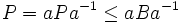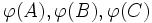# Three solvable subgroups of pairwise coprime indexes implies solvable

## Statement

Suppose$G$ is a finite group and$A,B,C$ are solvable subgroups of$G$, such that the indices$[G:A], [G:B], [G:C]$ are pairwise relatively prime. Then,$G$ is a finite solvable group.

## Proof

Given: A finite group$G$. Three solvable subgroups$A,B,C$ of$G$ such that$[G:A], [G:B], [G:C]$ are pairwise relatively prime.

To prove:$G$ is solvable.

Proof: We prove the claim by induction on the order of$G$. The main part of the induction step is demonstrating the existence of a nontrivial solvable normal subgroup inside$G$.

Note that for$G$ nontrivial, none of the three subgroups can be trivial, for that would contradict the assumption that the indices are relatively prime. Also, note that if any of the three subgroups equals the whole group, then the whole group is anyway solvable. So, we can restrict our attention to the case where all three subgroups are proper nontrivial solvable subgroups.

### Existence of a nontrivial solvable normal subgroup

1.$A$ has an elementary abelian nontrivial normal subgroup, say$P$, a$p$-group: Since$A$ is a finite group, it has a minimal normal subgroup. Fact (1) now yields that the minimal normal subgroup must be elementary abelian. Call this$P$. Let$p$ be the prime for which$P$ is a power of$p$.
2.$P$ is contained in some conjugate of either$B$ or$C$ (without loss of generality,$P \le B$): Since$[G:B]$ and$[G:C]$ are relatively prime, at least one of them must be relatively prime to$p$. Suppose$[G:B]$ is relatively prime to$p$. Then, by fact (2),$B$ has a$p$-Sylow subgroup$Q$, which by order considerations is also$p$-Sylow in$G$. Further, fact (3) yields that$P$ is contained in some conjugate of$Q$. In particular,$P$ is contained in some conjugate of$B$. Finally, since conjugate subgroups have the same index, we can replace$B$ with that conjugate to begin with, and thus assume without loss of generality that$P \le B$.
3.$AB = G$: This follows from the assumption that the indices are relatively prime.
4. The normal core$N$ of$B$ in$G$ contains$P$: Since$AB = G$, the intersection of all conjugates$gBg^{-1}, g \in G$, equals the intersection of all conjugates$aBa^{-1}, a \in A$. However, since$P$ is normal in$A$, we have that$aPa^{-1} = P$ for all$a \in A$. In particular,$P = aPa^{-1} \le aBa^{-1}$. Thus,$P$ is contained in the intersection of all$aBa^{-1}$, so$P$ is contained in the normal core of$B$ in$G$.
5. The normal core$N$ of$B$ in$G$ is a nontrivial solvable normal subgroup of$G$: By the previous step,$P \le N$, and$P$ itself is nontrivial, so$N$ is nontrivial. Since$N \le B$ by definition, and$B$ is solvable, fact (4) yields that$N$ is solvable. Finally, the definition of normal core yields that$N$ is normal in$G$.

### The induction step

We have thus established that any finite group$G$ containing three solvable subgroups$A,B,C$ of pairwise coprime indices has a nontrivial solvable normal subgroup$N$. Now, consider the quotient map$\varphi:G \to G/N$. Under this map,$\varphi(A), \varphi(B), \varphi(C)$ are solvable subgroups of$G/N$, and their indices continue to be pairwise relatively prime. Thus,$G/N$ is solvable by the induction hypothesis. We now use fact (5) to conclude that$G$ itself is solvable.